# Add One Resistor to Give Bipolar LM555 Oscillator a 50:50 Duty Cycle

## Texas Instruments LM555

More than a half-century old, the ubiquitous and mind-bogglingly useful 555 analog timer has become a perennial object for both kudus and criticism. Most of the former and some of the latter is justified, but sometimes a supposed shortcoming will grow to the status of “common knowledge” just because a simple fix has been overlooked, even for a part in such long, wide, and popular use as the 555. A case in point is the oft stated (yet mistaken) notion that it’s complicated to coax the original (bipolar) version of the 555 to oscillate with a symmetrical square wave 50:50% duty cycle.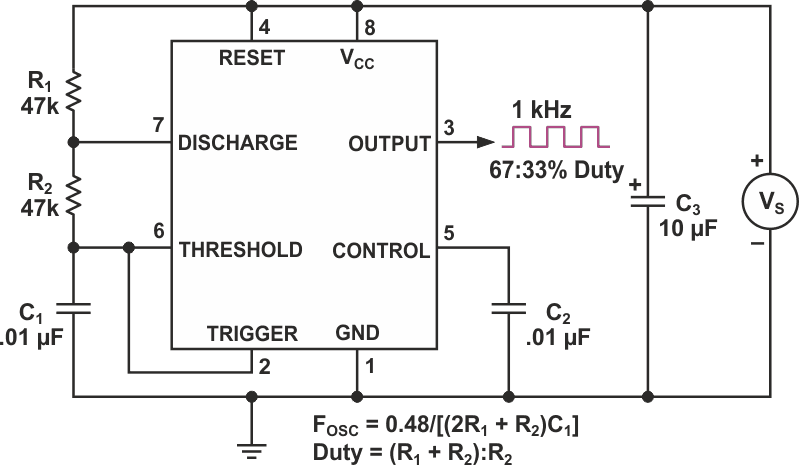Figure 1. «Traditional two-resistor» 555 oscillator.

The origin of this myth seems to be early 555 datasheet application notes that illustrated the topology shown in Figure 1 for astable oscillation. Its expression for output duty cycle, D, which is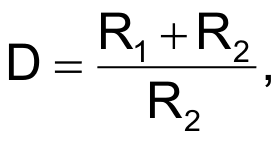tells us 50:50 will be impossible unless R1 = 0. Since a short circuit from DISCHARGE to VS would seem more likely to make the part output smoke than square waves, the conclusion follows: 50:50 square waves are, indeed, impossible.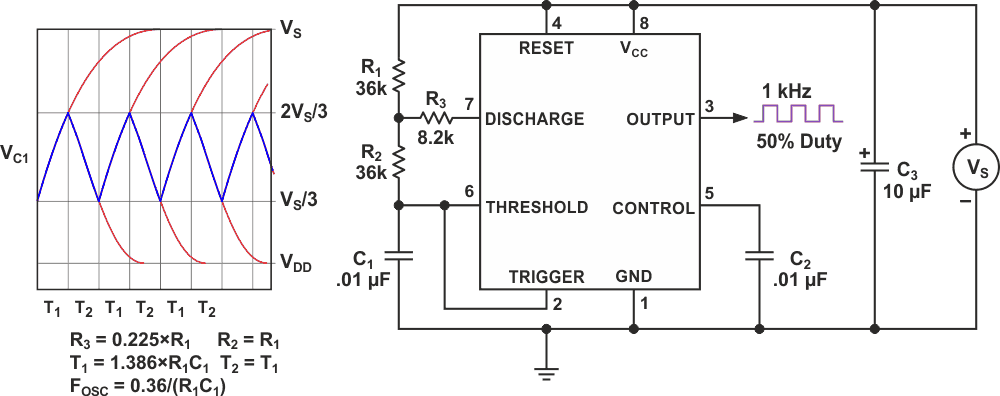Figure 2. New «three resistor» topology.

However, fortunately, as illustrated in Figure 2, the classic two-resistor topology isn’t the only way available to defrock this feline. Specifically, if a third resistor (R3) is added in series with the DISCHARGE pin, then a new signal level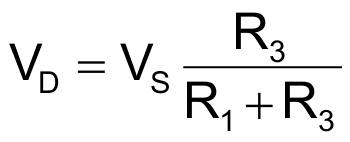is introduced as the asymptotic limit for the exponential discharge of C1, thus stretching the T2 phase of the oscillation cycle. In addition, the RC time constant for T2 is increased from R2C1 to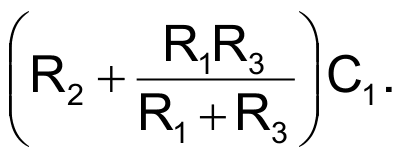The net effect, if R2 = R1 and R3 = 0.225•R1, then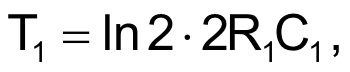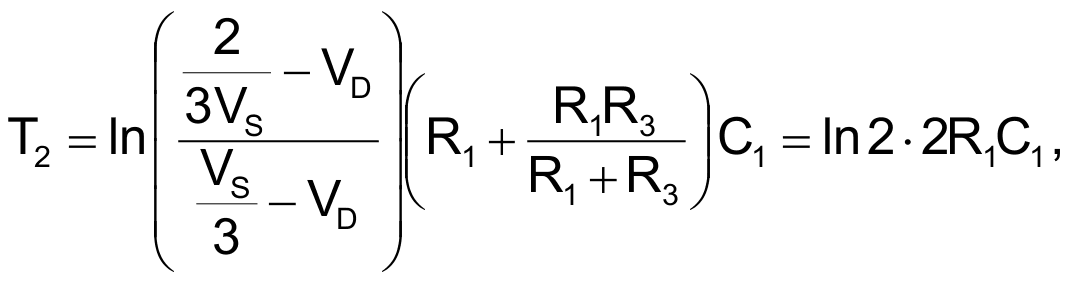T1 = T2,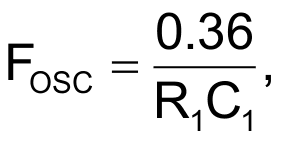D = 50:50.

The method also works for many R2/R1 ratios different from 1.0. Some examples appear in the Table 1:

Table 1.
 R2/R1 R3/R1 5.0 0.076 4.0 0.092 3.0 0.114 2.0 0.152 1.8 0.162 1.6 0.174 1.4 0.188 1.2 0.205 1.0 0.225 0.9 0.236 0.8 0.249 0.7 0.263 0.6 0.278 0.5 0.296 0.0 0.423

In every case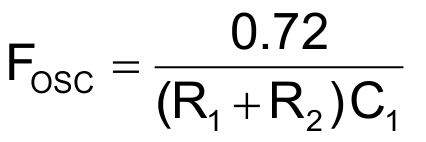and duty cycle = 50:50.

As noted, the method works all the way down to R2 = 0 (i.e., leaving only R1 and R3 with R2 replaced with a direct connection). However, R3 = 0.423•R1 makes VD = 0.297•VS which is uncomfortably close to the 555’s nominal TRIGGER voltage = 0.333•VS and may seriously exaggerate the effects of offset voltages and on-chip resistor tolerances on FOSC and duty cycle.

Meanwhile, what’s the moral to our story? Even after 50 years, myths are made for busting!

## Materials on the topic

EDN